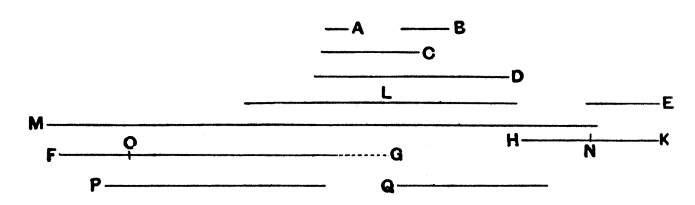#Elements: Book IX, Proposition 36

Math Lair Home > Source Material > Elements > Book IX, Proposition 36

The following is as given in Sir Thomas L. Heath's translation, which can be found in the book The Thirteen Books of The Elements, Vol. 2.

Elements on the Math Lair
Book IBook IIBook IX
Definitions, Postulates, and Common NotionsDefinitionsProposition 20
Proposition 1, Proposition 3, Proposition 14Proposition 36
Proposition 5, Proposition 6,
Proposition 29, Proposition 47

## Proposition 36.

If as many numbers as we please beginning from an unit be set out continuously in double proportion, until the sum of all becomes prime, and if the sum multiplied into the last make some number, the product will be perfect.

For let as many numbers as we please, A, B, C, D, beginning from an unit be set out in double proportion, until the sum of all becomes prime,

let E be equal to the sum, and let E by multiplying D make FG;
I say that FG is perfect.

For, however many A, B, C, D are int multitude, let so many E, HK, L, M be taken in double proportion beginning from E;

therefore, ex aequali, as A is to D, so is. E to M. [VII. 14]

Therefore the product of E, D is FG;

therefore the product of A, M is also FG.

Therefore A by multiplying M has made FG;
therefore M measures FG according to the units in A.

therefore FG is double of M.But M, L, HK, E are continuously double of each other; therefore E, HK, L, M, FG are continuously proportional in double proportion.

Now let there be subtracted from the second HK and the last FG the numbers HN, FO, each equal to the first E; therefore, as the excess of the second is to the first, so is the excess of the last to all those before it. [IX. 35]

Therefore, as NK is to E, so is OG to M, L, KH, E.

And NK is equal to E;

therefore OG is also equal to M, L, KH, E.

But FO is also equal to E,

and E is equal to A, B, C, D and the unit.

Therefore the whole FG is equal to E, HK, L, M and A, B, C, D and the unit.

and it is measured by them.

I say also that FG will not be measured by any other number except A, B, C, D, E, HK, L, M and the unit.

For, if possible, let some number P measure FG,

and let P not be the same with any of the numbers A, B, C, D, E, HK, L, M.

And, as many times as P measures FG, so many units let there be in Q;

therefore Q by multiplying P has made FG.

But, further, E has also by multiplying D made FG; therefore, as E is to Q, so is P to D.

And, since A, B, C, D are continuously proportional beginning from an unit,

therefore D will not be measured by any other number except A, B, C. [IX. 13]

And, by hypothesis, P is not the same with any of the numbers A, B, C;

therefore P will not measure D.

But, as P is to D, so is E to Q;

therefore, neither does E measure Q. [VII. Def. 20]

And E is prime;

and any prime number is prime to any number which it does not measure. [VII. 29]

Therefore E, Q are prime to one another.

But primes are also least, [VII. 21]

and the least numbers measure those which have the same ratio the same number of times, the antecedent the antecedent and the consequent the consequent; [VII. 20]
and, as E is to Q, so is P to D;
therefore E measures P the same number of times that Q measures D.

But D is not measured by any number except A, B, C;

therefore Q is the same with one of the numbers A, B, C.

Let it be the same with B.

And, however many B, C, D are in multitude, let so many E, HK, L be taken beginning from E.

Now E, HK, L are in the same ratio with B, C, D;

therefore, ex aequali, as B is to D, so is E to L. [VII. 14]

Therefore the product of B, L is equal to the product of D, E. [VII. 19]

But the product of D, E is equal to the product of Q, P; therefore the product of Q, P is also equal to the product of B, L.

Therefore, as Q is to B, so is L to P. [VII. 19]

And Q is the same with B;

therefore L is also the same with P;
which is impossible, for by hypothesis P is not the same with any of the numbers set out.

Therefore no number will measure FG except A, B, C, D, E, HK, L, M and the unit.

And FG was proved equal to A, B, C, D, E, HK, L, M and the unit;

and a perfect number is that which is equal to its own parts; [VII. Def. 22]
therefore FG is perfect.

Q.E.D.